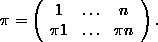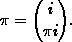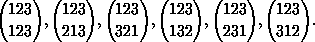Finite symmetric groups

## Finite symmetric groups

In the first section we mentioned the symmetric group SX on the set X. In order to prepare further examples and detailed descriptions of actions we need to consider this group in some detail, in particular for finite X. A first remark shows that it is only the order of X which really matters:
Lemma: For any two finite and nonempty sets X and Y, the natural actions of SX on X and SY on Y are isomorphic if and only if | X | = | Y | .

This is very easy to check and therefore left as an exercise. We call | X | the degree of SX, of any subgroup P £SX and of any pÎSX. In order to examine permutations of degree n it therefore suffices to consider a particular set of order n and its symmetric group. For technical reasons we introduce two such sets of order n:

n:= {0, ...,n-1 } and n:= {1, ...,n },
hoping that it will be always clear from the context if this set n is meant or its cardinality n. It is an old tradition to prefer the set n and its symmetric group which we should denote by Sn in order to be consistent. Hence let us fix the notation for the elements of Sn , the corresponding notation for the elements of Sn is then obvious. A permutation pÎSn is written down in full detail by putting the images pi in a row under the points i În, sayThis will be abbreviated byHence, for example, S 3 consists of the following elements:In our programs permutations will be written in the form [ p1, p2, ..., pn]. There is a program to compute all elements of the symmetric group Sn .
• Cycle decomposition
• Conjugation
• Conjugacy Classes
• Generators of the symmetric group
• Subgroups of Cyclic Groups
• Colourings of the n Gon
• The Sign
• Splitting Orbits
• Rothe diagram and inversions
• The Lehmer code and reduced decompositions
• Sn as a Coxeter Group
• The Exchange Lemma
• Exercises

• harald.fripertinger@kfunigraz.ac.at,
last changed: August 28, 2001Finite symmetric groups